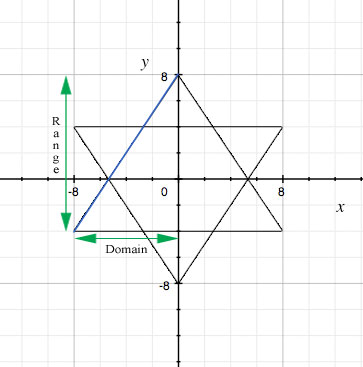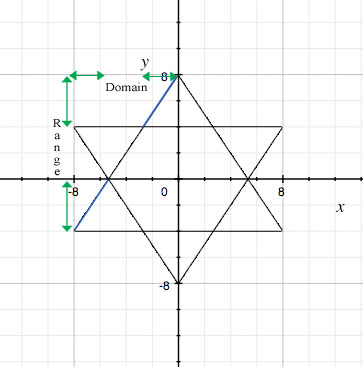SEARCH HOMEMath Central Quandaries & QueriesDraw the Star to scale on a piece of graph paper. In this case I used a 12 unit vertical and a 12 unit horizontal scale. He must find the equation, the domain and the range for each line. If you can help me with the domain and range for one of lines, I will do the rest. Example: line 1 y = mx+b b=8 and m=(12/8) or 1.5 so y=1.5x+8 I'm not sure of the domain & range. I think the domain would be all real numbers from -8 to 0 and the range would be all real numbers from -4 to 8 but it has been just too many years. Thanks.Hi Larry,

Domain is the all the possible x values and range is all of the possible y values. I think I reproduced the star correctly. I think there are a couple ways to interpret what part of the line you need to find the domain and range for. I highlighted the line I am referring to in blue.If you take the line to be the whole side, then domain: x∈(-8,0) (all real numbers between -8 & 0) range: y∈(-8,8)If you take the line to only be the edges of the star, then domain: x∈(-8,-5 1/3) and (5 1/3, 8) range: y∈(-4, 0) and (4,8)

Hope this helps,

JaniceMath Central is supported by the University of Regina and The Pacific Institute for the Mathematical Sciences.